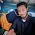## 20.7.13

### C++ : Interfaces

The C++ interfaces are implemented using abstract classes and these abstract classes should not be confused with data abstraction which is a concept of keeping implementation detail separate from associated data.
A class is made abstract by declaring at least one of its functions as pure virtual function. A pure virtual function is specified by placing "= 0" in its declaration as follows:
```class Box
{
public:
// pure virtaul function
virtual double getVolume() = 0;
private:
double length;      // Length of a box
double breadth;     // Breadth of a box
double height;      // Height of a box
};```
The purpose of an abstract class (often referred to as an ABC) is to provide an appropriate base class from which other classes can inherit. Abstract classes cannot be used to instantiate objects and serves only as an interface. Attempting to instantiate an object of an abstract class causes a compilation error.
Thus, if a subclass of an ABC needs to be instantiated, it has to implement each of the virtual functions, which means that it supports the interface declared by the ABC. Failure to override a pure virtual function in a derived class, then attempting to instantiate objects of that class, is a compilation error.
Classes that can be used to instantiate objects are called concrete classes.

## Abstract Class Example:

Consider the following example where parent class provides an interface to the base class to implement a function called getArea():
```#include <iostream>

using namespace std;

// Base class
class Shape
{
public:
// pure virtual function providing interface framework.
virtual int getArea() = 0;
void setWidth(int w)
{
width = w;
}
void setHeight(int h)
{
height = h;
}
protected:
int width;
int height;
};

// Derived classes
class Rectangle: public Shape
{
public:
int getArea()
{
return (width * height);
}
};
class Triangle: public Shape
{
public:
int getArea()
{
return (width * height)/2;
}
};

int main(void)
{
Rectangle Rect;
Triangle  Tri;

Rect.setWidth(5);
Rect.setHeight(7);
// Print the area of the object.
cout << "Total Rectangle area: " << Rect.getArea() << endl;

Tri.setWidth(5);
Tri.setHeight(7);
// Print the area of the object.
cout << "Total Triangle area: " << Tri.getArea() << endl;

return 0;
}```
When the above code is compiled and executed, it produces following result:
```Total Rectangle area: 35
Total Triangle area: 17```
You can see how an abstract class defined an interface in terms of getArea() and two other classes implemented same function but with different algorithm to calculate the area specific to the shape.

#### 1 comment:

1.Abstract Class Example:
Consider the following example where parent class provides an interface to the base class to implement a function called getArea():

#include

using namespace std;

// Base class
class Shape
{
public:
// pure virtual function providing interface framework.
virtual int getArea() = 0;
void setWidth(int w)
{
width = w;
}
void setHeight(int h)
{
height = h;
}
protected:
int width;
int height;
};

// Derived classes
class Rectangle: public Shape
{
public:
int getArea()
{
return (width * height);
}
};
class Triangle: public Shape
{
public:
int getArea()
{
return (width * height)/2;
}
};

int main(void)
{
Rectangle Rect;
Triangle Tri;

Rect.setWidth(5);
Rect.setHeight(7);
// Print the area of the object.
cout << "Total Rectangle area: " << Rect.getArea() << endl;

Tri.setWidth(5);
Tri.setHeight(7);
// Print the area of the object.
cout << "Total Triangle area: " << Tri.getArea() << endl;

return 0;
}

When the above code is compiled and executed, it produces following result:

Total Rectangle area: 35
Total Triangle area: 17

Comment Here